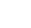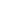# Covariance and Correlation In Machine Learning

0
1953## IntroductionCovariance and Correlation

Covariance and correlation both are mathematical concepts that are also used in statistics and probability theory. Most useful in understanding variables. Generally use the data science field for comparing data samples from different populations, and covariance is used to determine how much two random variables to each other, whereas correlation, is used to determine change one variable is it affect another variable.

Both term are related to the linear relationship between variables. In another word, if one variable goes to increasing direction the same as another variable goes that direction, it means a positive correlation. If both variable are in the opposite direction then called negative correlation.

When there is no relationship, there is no any changes. Correlation explains the change in one variable leads to how much change in the second variable. Read more about How Tesla use AI & CV.

### Sample

A sample is randomly chosen from population. We calculate covariance and correlation on samples rather than the complete population.

## Covariance

Covariance is only dependent upon sign. A positive value shows both variables in the same direction. Same as A negative value shows both are in opposite direction. Covariance is a measured use to determine how much variable change in randomly. The covariance is a product of the units of the two variables. The value of covariance lies between -∞ and +∞. The covariance of two variables (x and y) can be represented by cov(x,y).E[x] is the expected value or also called as means of sample ‘x’.

Where,

• x̄ = sample mean of x
• ȳ = sample mean of y
• x_i and y_i = the values of x and y for ith record in the sample.
• N =  is the no of records in the sample

## Significance of the formula

• Numerator show, the quantity of variance in x multiplied by the quantity of variance in y.
• Unit of covariance shows, Unit of x multiplied by a unit of y
• Hence if we change the unit of variables, covariance also has new value but sign will remain the same.
• However if it is positive then both variables vary in the same direction else if it is negative then they vary in the opposite direction.

## Correlations

Correlation means, correlation between two variables which is a normalized version of the covariance. The range of correlation coefficients is always between -1 to 1. The correlation coefficient is also known as Pearson’s correlation coefficient. As you read about Covariance it only tells about the direction but which is not enough to understand the relationship completely. So, we divide the covariance with a standard deviation of x and y respectively.

The correlation coefficient between the random variables X and Y, you have to divide the sample covariance of X and Y by the product of the sample sat.deviation of X and Y respectively.

## Significance

• -1 and +1 indicate that both variables have a perfect linear relationship.
• Negative means they are inversely proportional to each other with the factor of correlation coefficient value.
• Positive means they are directly proportional to each other mean vary in the same direction with the factor of correlation coefficient value.
• if the correlation coefficient is 0 then it means there is no linear relationship between variables.

Where,

• Correlation = sample correlation between X and Y
•  Cov(X,Y) = sample covariance between X and Y
•= sample standard deviation of X
•= sample standard deviation of Y

## Difference Between Covariance and Correlation

Correlation is simply a normalized form of covariance. It is obviously important to be precise with language when discussing the two, but conceptually they are almost identical.

The value of the correlation coefficient ranges from [-1 – 1]. -1 is indicate for a negative relationship. 1 means a positive relationship. 0 means no relationship.

To get a sense of what correlated data looks like let us plot two correlated datasets

## Implementation

#import necessary library

``````import os
import sys
import numpy as np
import matplotlib.pyplot as plt
import seaborn as sns

X = np.random.rand(50)       #random number
Y = 2 * X + np.random.normal(0, 0.1, 50)

#covariance
cov_matrix = np.cov(X, Y)      #calculate covariance between x & y
print('The Covariance of X and Y: %.2f'%cov_matrix[0, 1])

The covariance of X and Y: 0.21

#Correlation

cor_matrix = np.corrcoef(X, Y) #calculate correlation between x & y

print(Correlation of X and Y: %.2f'%cor_matrix[0, 1])

Correlation of X and Y: 0.99
``````

## Conclusion

Both covariance and correlation measure the linear relationship between variables but cannot be used interchangeably. Want to start your career in the field of data science? Visit our website to know how you can start our journey with our job oriented programs